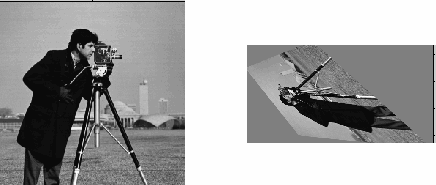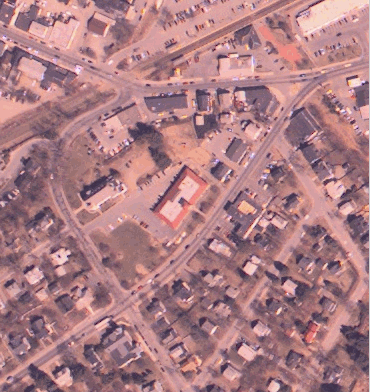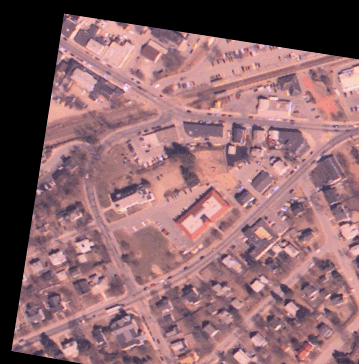# imtransform

(Not recommended) Apply 2-D spatial transformation to image

`imtransform` is not recommended. Use `imwarp` instead for 2-D and 3-D transformations. Use `tformarray` for higher dimensional transformations.

## Syntax

``B = imtransform(A,tform)``
``B = imtransform(A,tform,interp)``
``B = imtransform(___,Name,Value)``
``[B,xdata,ydata] = imtransform(___)``

## Description

example

````B = imtransform(A,tform)` transforms image `A` according to the 2-D spatial transformation defined by `tform`, and returns the transformed image, `B`.If `A` is a color image, then `imtransform` applies the same 2-D transformation to each color channel. Likewise, if `A` is a volume or image sequence with three or more dimensions, then `imtransform` applies the same 2-D transformation to all 2-D planes along the higher dimensions.```
````B = imtransform(A,tform,interp)` specifies the form of interpolation to use.```
````B = imtransform(___,Name,Value)` uses name-value pairs to control various aspects of the spatial transformation.```

example

````[B,xdata,ydata] = imtransform(___)` also returns the extent of the output image `B` in the output X-Y space. By default, `imtransform` calculates `xdata` and `ydata` automatically so that `B` contains the entire transformed image `A`. However, you can override this automatic calculation by specifying values for the `XData` and `YData` name-value pair input arguments.```

## Examples

### Simple Transformation

Apply a horizontal shear to a grayscale image.

```I = imread('cameraman.tif'); tform = maketform('affine',[1 0 0; .5 1 0; 0 0 1]); J = imtransform(I,tform); imshow(J)```### Projective Transformation

Map a square to a quadrilateral with a projective transformation. Set up an input coordinate system so that the input image fills the unit square with vertices (0 0), (1 0), (1 1), (0 1).

```I = imread('cameraman.tif'); udata = [0 1]; vdata = [0 1];```

Transform to a quadrilateral with vertices (-4 2), (-8 3), (-3 -5), (6 3).

```tform = maketform('projective',[ 0 0; 1 0; 1 1; 0 1],... [-4 2; -8 -3; -3 -5; 6 3]);```

Fill with gray and use bicubic interpolation. Make the output size the same as the input size.

```[B,xdata,ydata] = imtransform(I,tform,'bicubic', ... 'udata',udata,... 'vdata',vdata,... 'size',size(I),... 'fill',128); subplot(1,2,1); imshow(I,'XData',udata,'YData',vdata) subplot(1,2,2); imshow(B,'XData',xdata,'YData',ydata)```### Image Registration

Read an aerial photo into the MATLAB® workspace and view it.

```unregistered = imread('westconcordaerial.png'); figure imshow(unregistered)```Read an orthophoto into the MATLAB workspace and view it.

```figure imshow('westconcordorthophoto.png')```Load control points that were previously picked.

`load westconcordpoints`

Create a transformation structure for a projective transformation using the points.

`t_concord = cp2tform(movingPoints,fixedPoints,'projective');`

Get the width and height of the orthophoto, perform the transformation, and view the result.

```info = imfinfo('westconcordorthophoto.png'); registered = imtransform(unregistered,t_concord,... 'XData',[1 info.Width],'YData',[1 info.Height]); figure imshow(registered)```## Input Arguments

collapse all

Image to be transformed, specified as a numeric or logical array of any dimension.

Data Types: `single` | `double` | `int8` | `int16` | `int32` | `int64` | `uint8` | `uint16` | `uint32` | `uint64` | `logical`

Transformation structure, specified as a `TFORM` structure, such as returned by `maketform`. The first dimension of the transformation is the horizontal or x-coordinate, and the second dimension is the vertical or y-coordinate. This convention is the reverse of the array subscripting convention in MATLAB.

Interpolation method, specified as one of these values.

Interpolation MethodDescription
`'bilinear'`Linear interpolation
`'nearest'`Nearest-neighbor interpolation—the output pixel is assigned the value of the pixel that the point falls within. No other pixels are considered.
`'bicubic'`Cubic interpolation
`resampler` structure`resampler` structure returned by `makeresampler`. This option allows more control over how `imtransform` performs resampling.

Data Types: `char`

### Name-Value Arguments

Specify optional comma-separated pairs of `Name,Value` arguments. `Name` is the argument name and `Value` is the corresponding value. `Name` must appear inside quotes. You can specify several name and value pair arguments in any order as `Name1,Value1,...,NameN,ValueN`.

Example: `'FillValues',128`

Spatial extent of input image `A` in the U-V input space, specified as 2-element numeric vectors. The values of `UData` and `VData` represent coordinates in the world coordinate system. The two elements of `UData` give the u-coordinates (horizontal) of the first and last columns of `A`, respectively. The two elements of `VData` give the v-coordinates (vertical) of the first and last rows of `A`.

By default, the spatial extent of `A` in the U-V space is the same as the image extent in intrinsic coordinates. In other words, the default value of `UData` is `[1 size(A,2)]` and the default value of `VData` is ```[1 size(A,1)]```.

Spatial extent of transformed image `B` in the X-Y input space, specified as 2-element numeric vectors. The values of `XData` and `YData` represent coordinates in the world coordinate system. The two elements of `XData` give the x-coordinates (horizontal) of the first and last columns of `B`, respectively. The two elements of `YData` give the y-coordinates (vertical) of the first and last rows of `B`.

If you do not specify `XData` and `YData`, then `imtransform` estimates values that contain the entire transformed output image. To determine these values, `imtransform` uses the `findbounds` function.

Size of pixels in X-Y output space, specified as a numeric scalar or 2-element numeric vector. If `XYScale` is a scalar, then output pixels are square and `XYScale` specifies the side length. Otherwise, the two elements of `XYScale` specify the width and height of each output pixel in X-Y space, respectively.

The default value of `XYScale` depends on whether you specify `Size`:

• If you specify `Size`, then `imtransform` calculates `XYScale` from `Size`, `XData`, and `YData`.

• If you do not specify `Size`, then `imtransform` uses the scale of the input pixels for `XYScale`, except in cases where an excessively large output image would result.

Note

In cases where preserving the scale of the input image would result in an excessively large output image, the `imtransform` function automatically increases the value of `XYScale`. To ensure that the output pixel scale matches the input pixel scale, specify the `XYScale` parameter. For example, call `imtransform` as shown in the following syntax:

`B = imtransform(A,T,'XYScale',1)`

Size of transformed image `B`, specified as a 2-element vector of positive integers. The two elements of `Size` specify the number of rows and columns of the output image `B`, respectively. For higher dimensions, `imtransform` takes the size of `B` directly from the size of input image `A`. Thus, `size(B,k)` equals `size(A,k)` for `k > 2`.

If you do not specify `Size`, then `imtransform` derives this value from `XData`, `YData`, and `XYScale`.

Fill value used for output pixels outside the input image boundaries, specified as the comma-separated pair consisting of `'FillValues'` and a numeric scalar or numeric array. Fill values are used for output pixels when the corresponding inverse transformed location in the input image is completely outside the input image boundaries.

• If the input image `A` is 2-D, then `FillValues` must be a scalar.

• If `A` is 3-D or N-D, then `FillValues` can be an array whose size satisfies the following constraint: `size(FillValues,k)` must equal either `size(A,k+2)` or `1`.

For example, if `A` is a `uint8` RGB image that is 200-by-200-by-3, then possibilities for `'FillValues'` include the following values.

ValueFill
`0`Fill with black
`[0;0;0]`Fill with black
`255`Fill with white
`[255;255;255]`Fill with white
`[0;0;255]`Fill with blue
`[255;255;0]`Fill with yellow

For a second example, if `A` is 4-D with size 200-by-200-by-3-by-10, then you can specify `'FillValues'` as a scalar, 1-by-10 vector, 3-by-1 vector, or 3-by-10 matrix.

## Output Arguments

collapse all

Transformed image, returned as a numeric or logical array of the same dimensionality as the input image `A`.

Horizontal extent of the transformed image `B` in X-Y output space, returned as a 2-element numeric vector. The two elements of `xdata` give the x-coordinates (horizontal) of the first and last columns of `B` in the world coordinate system, respectively.

Note

The first element of `xdata` always equals the first element of the `XData` argument, if specified. However, sometimes the second element of `xdata` does not exactly equal the second element of `XData`. The values differ either because of the need for an integer number of rows and columns, or because you specified values for `XData`, `YData`, `XYScale`, and `Size` that are not entirely consistent.

Vertical extent of the transformed image `B` in X-Y output space, returned as a 2-element numeric vector. The two elements of `ydata` give the y-coordinates (vertical) of the first and last rows of `B` in the world coordinate system, respectively.

Note

The first element of `ydata` always equals the first element of the `YData` argument, if specified. However, sometimes the second element of `ydata` does not exactly equal the second element of `YData`. The values differ either because of the need for an integer number of rows and columns, or because you specified values for `XData`, `YData`, `XYScale`, and `Size` that are not entirely consistent.

## Tips

• Image Registration. The `imtransform` function automatically shifts the origin of your output image to make as much of the transformed image visible as possible. If you use `imtransform` to do image registration, the syntax ```B = imtransform(A,tform)``` can produce unexpected results. To control the spatial location of the output image, set `XData` and `YData` explicitly.

• Pure Translation. Calling the `imtransform` function with a purely translational transformation results in an output image that is exactly like the input image unless you specify `XData` and `YData` values in your call to `imtransform`. For example, if you want the output to be the same size as the input revealing the translation relative to the input image, call `imtransform` as shown in the following syntax:

```B = imtransform(A,T,'XData',[1 size(A,2)],... 'YData',[1 size(A,1)])```

• Transformation Speed. If you do not specify the output-space location for `B` using `XData` and `YData`, then `imtransform` estimates the location automatically using the function `findbounds`. You can use `findbounds` as a quick forward-mapping option for some commonly used transformations, such as affine or projective. For transformations that do not have a forward mapping, such as polynomial transformations computed by `fitgeotrans`, `findbounds` can take much longer. If you can specify `XData` and `YData` directly for such transformations, then `imtransform` may run noticeably faster.

• Clipping. The automatic estimate of `XData` and `YData` using `findbounds` sometimes clips the output image. To avoid clipping, set `XData` and `YData` directly.